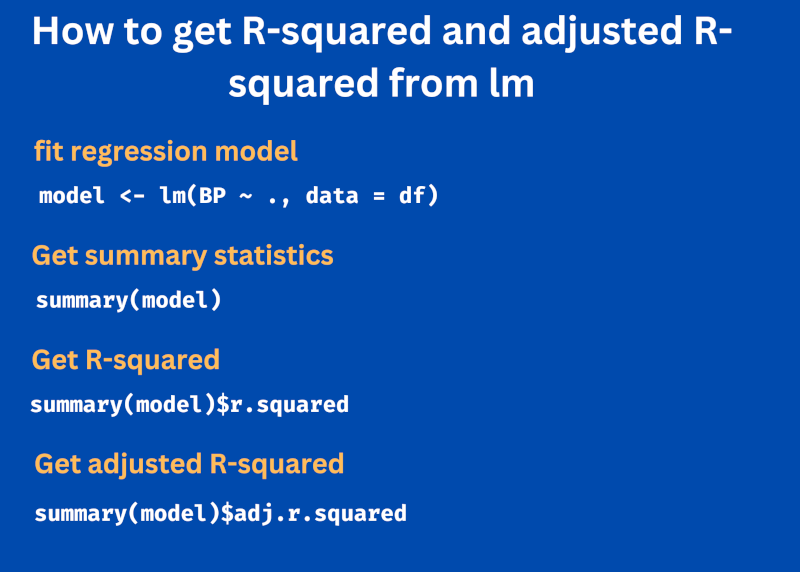# How to Get R-Squared and Adjusted R-Squared From `lm` in RWhen you perform a regression analysis using the `lm()` function, you can get the summary statistics of regression model using the `summary()` function.

The summary statistics give detailed information for fitted regression model including model formula, regression coefficients, residuals, and other statistical information such as standard error and R-Squared.

Sometimes, we are interested to only getting R-Squared and adjusted R-Squared values instead of getting detailed summary statistics.

You can use the following syntax to get R-Sqaured and adjusted R-Squared from summary statistics.

``````# R-Sqaured
summary(model)\$r.squared

``````

The multiple regression example below illustrates how to get the R-Squared and adjusted R-Squared values based on the fitted model.

Load blood pressure example dataset to fit the regression model,

``````df = read.csv("https://reneshbedre.github.io/assets/posts/reg/bp.csv")

# view first 5 rows
BP Age Weight  BSA Dur Pulse Stress
1 105  47   85.4 1.75 5.1    63     33
2 115  49   94.2 2.10 3.8    70     14
3 116  49   95.3 1.98 8.2    72     10
4 117  50   94.7 2.01 5.8    73     99
5 112  51   89.4 1.89 7.0    72     95
6 121  48   99.5 2.25 9.3    71     10
``````

Fit the multiple regression model using the `lm()` function with `BP` as a dependent variable,

``````model <- lm(BP ~ ., data = df)

# summary statistics
summary(model)

# output
Call:
lm(formula = BP ~ ., data = df)

Residuals:
Min       1Q   Median       3Q      Max
-0.93213 -0.11314  0.03064  0.21834  0.48454

Coefficients:
Estimate Std. Error t value Pr(>|t|)
(Intercept) -12.870476   2.556650  -5.034 0.000229 ***
Age           0.703259   0.049606  14.177 2.76e-09 ***
Weight        0.969920   0.063108  15.369 1.02e-09 ***
BSA           3.776491   1.580151   2.390 0.032694 *
Dur           0.068383   0.048441   1.412 0.181534
Pulse        -0.084485   0.051609  -1.637 0.125594
Stress        0.005572   0.003412   1.633 0.126491
---
Signif. codes:  0 ‘***’ 0.001 ‘**’ 0.01 ‘*’ 0.05 ‘.’ 0.1 ‘ ’ 1

Residual standard error: 0.4072 on 13 degrees of freedom
Multiple R-squared:  0.9962,	Adjusted R-squared:  0.9944
F-statistic: 560.6 on 6 and 13 DF,  p-value: 6.395e-15
``````

The summary statistics provide values of R-squared (0.9962) and adjusted R-squared (0.9944) along with other regression model statistics. The R-squared and adjusted R-squared are used for checking the regression model performance.

The adjusted R-squared value helps interpret the performance of the multiple regression model as it corrects for sample size and regression coefficients.

If you only want to get R-squared and adjusted R-squared from summary statistics, you can extract those values using the `\$` operator.

Extract R-squared,

``````# R-Sqaured
summary(model)\$r.squared
0.9961503
``````

``````summary(model)\$adj.r.squared
0.9943734
``````